Processing ......FreeComputerBooks.com Links to Free Computer, Mathematics, Technical Books all over the World

Model-Theoretic Logics

• Title Model-Theoretic Logics
• Authors Jon Barwise (Editor), Solomon Feferman (Editor)
• Publisher: Cambridge University Press; 1 edition (March 2, 2017)
• Hardcover: 911 pages
• eBook: PDF, PostScript, DVI
• Language: English
• ISBN-10: 1107168252
• ISBN-13: 978-1107168251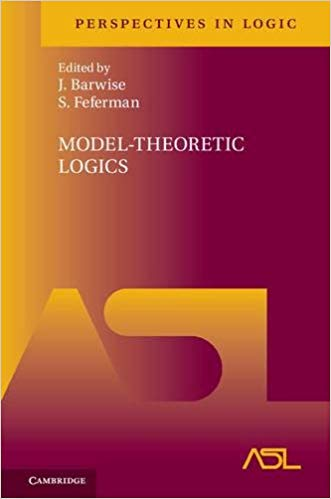Book Description

This book brings together several directions of work in model theory between the late 1950s and early 1980s. It contains expository papers by pre-eminent researchers. Part I provides an introduction to the subject as a whole, as well as to the basic theory and examples. The rest of the book addresses finitary languages with additional quantifiers, infinitary languages, second-order logic, logics of topology and analysis, and advanced topics in abstract model theory. Many chapters can be read independently.

• Jon Barwise was a mathematician, philosopher and logician. He taught at the Universities of Yale and Wisconsin before becoming the first director of the Center for the Study of Language and Information at Stanford University. From 1990 until his death he was professor of philosophy at Indiana University.
• Solomon Feferman was an American philosopher and mathematician with works in mathematical logic.
Reviews, Ratings, and Recommendations: Related Book Categories: Read and Download Links:Similar Books:
•Language, Proof and Logic (Jon Barwise, et al)

This book covers first-order language in a method appropriate for first and second courses in logic, and is specially useful to undergraduates of philosophy, computer science, mathematics, and linguistics.

•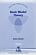Basic Model Theory (Kees Doets)

As the title indicates, this book introduces the reader to what is basic in model theory. A special feature is its use of the Ehrenfeucht game by which the reader is familiarised with the world of models.

•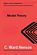Model Theory (C. Ward Henson)

It is an up-to-date textbook of model theory taking the reader from first definitions to Morley's theorem and the elementary parts of stability theory, introduces the model theory of first-order logic, avoiding syntactical issues not too relevant to model theory.

•Model Theory, Algebra, and Geometry (Deirdre Haskell, et al)

Tis book gives the necessary background for understanding both the model theory and the mathematics behind the apps, begins with an introduction to model theory, broadens into three components: pure model theory, geometry, and the model theory of fields.

•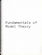Fundamentals of Model Theory (William Weiss, et al)

This book is a concluding discussion focuses on the relationship between proofs and formal derivations, and the role proofs may play as part of a general theory of evidence. It is a primer which will give someone a self contained overview of the subject.

•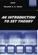An Introduction to Set Theory (William A. R. Weiss)

This book covers the basics: relations, functions, orderings, finite, countable, and uncountable sets, and cardinal and ordinal numbers, gives students sufficient grounding in a rigorous approach to the revolutionary results of set theory.

Book Categories
 :All CategoriesRecent BooksMiscellaneous BooksComputer LanguagesComputer ScienceData Science/DatabasesElectrical EngineeringJava and Java EE (J2EE)Linux and UnixMathematicsMicrosoft and .NETMobile ComputingNetworking and CommunicationsSoftware EngineeringSpecial TopicsWeb Programming
Other Categories